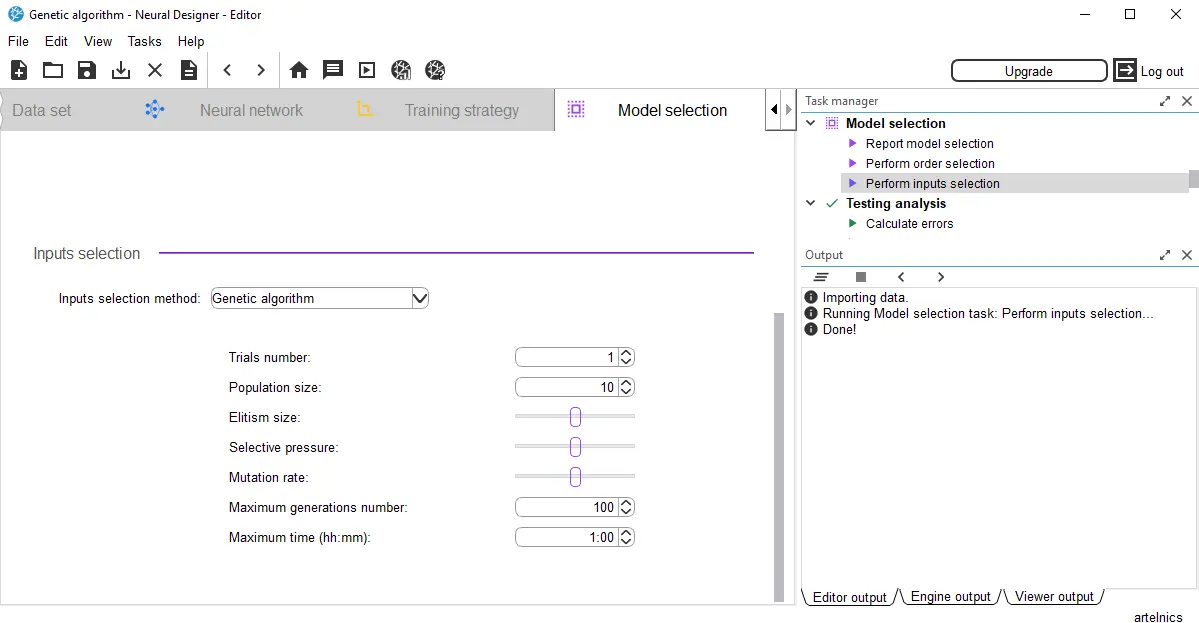﻿ Genetic algorithms for feature selection | Neural Designer

# Genetic algorithms for feature selectionMany typical machine learning applications, from customer targeting to medical diagnosis, arise from complex relationships between features (also-called input variables or characteristics).

Feature selection, or input selection algorithms search for the most relevant inputs for a model.

Feature selection techniques help identify and remove unneeded, irrelevant, and redundant features. Indeed, that variables do not contribute to or decrease the accuracy of the predictive model.

Contents:

## Feature selection

Mathematically, we formulate inputs selection as a combinatorial optimization problem.

The objective function is the predictive model's generalization performance, represented by the error term on the selection instances of a data set.

The design variables are the inclusion (1) or the exclusion (0) of the input variables in the neural network.

A wide selection of features would evaluate 2N different combinations, where N is the number of features.

This process requires lots of computational work, and it becomes impracticable if the number of features is significant. Therefore, we need intelligent methods to select features in practice.

## Genetic algorithms

One of the most advanced algorithms for feature selection is the genetic algorithm.

The genetic algorithm is a stochastic method for function optimization based on natural genetics and biological evolution.

In nature, organisms' genes tend to evolve over successive generations to better adapt to the environment. The genetic algorithm is a heuristic optimization method inspired by the procedures of natural evolution.

Genetic algorithms operate on a population of individuals to produce better and better approximations.

The algorithm creates a new population every generation by selecting individuals according to their fitness level in the problem domain. These individuals are then recombined together using operators borrowed from natural genetics. The offspring might also undergo mutation.

A state diagram for the feature selection process with the genetic algorithm is depicted next.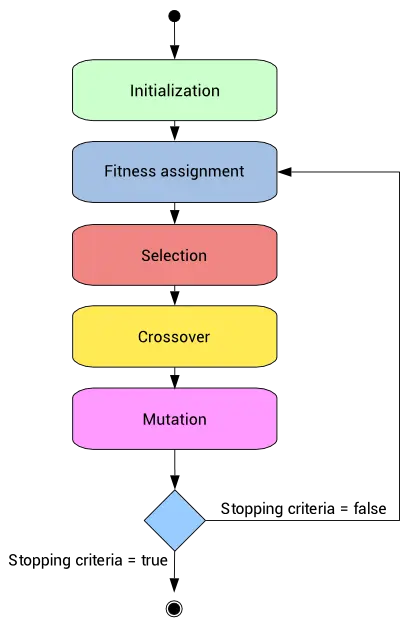This process leads to the evolution of populations better suited to their environment than the individuals who originated it.

In our case, each individual in the population represents a neural network.

Genes here are binary values, and represent the inclusion or not of particular features in the model. The number of genes is the total number of input variables in the data set.

The number of individuals, or population size, must be chosen for each application. The default value for the population size is 10 N, where N is the number of features.

Next, we describe the operators and the corresponding parameters used by the genetic algorithm in detail.

## 1. Initialization operator

The first step is to create and initialize the individuals in the population. Since the genetic algorithm is a stochastic optimization method, we randomly initialize the genes of all individuals.

To illustrate this operator, consider a predictive model represented by a neural network with 6 possible features. If we generate four individuals, we have four different neural networks with random features.

The next figure illustrates this population.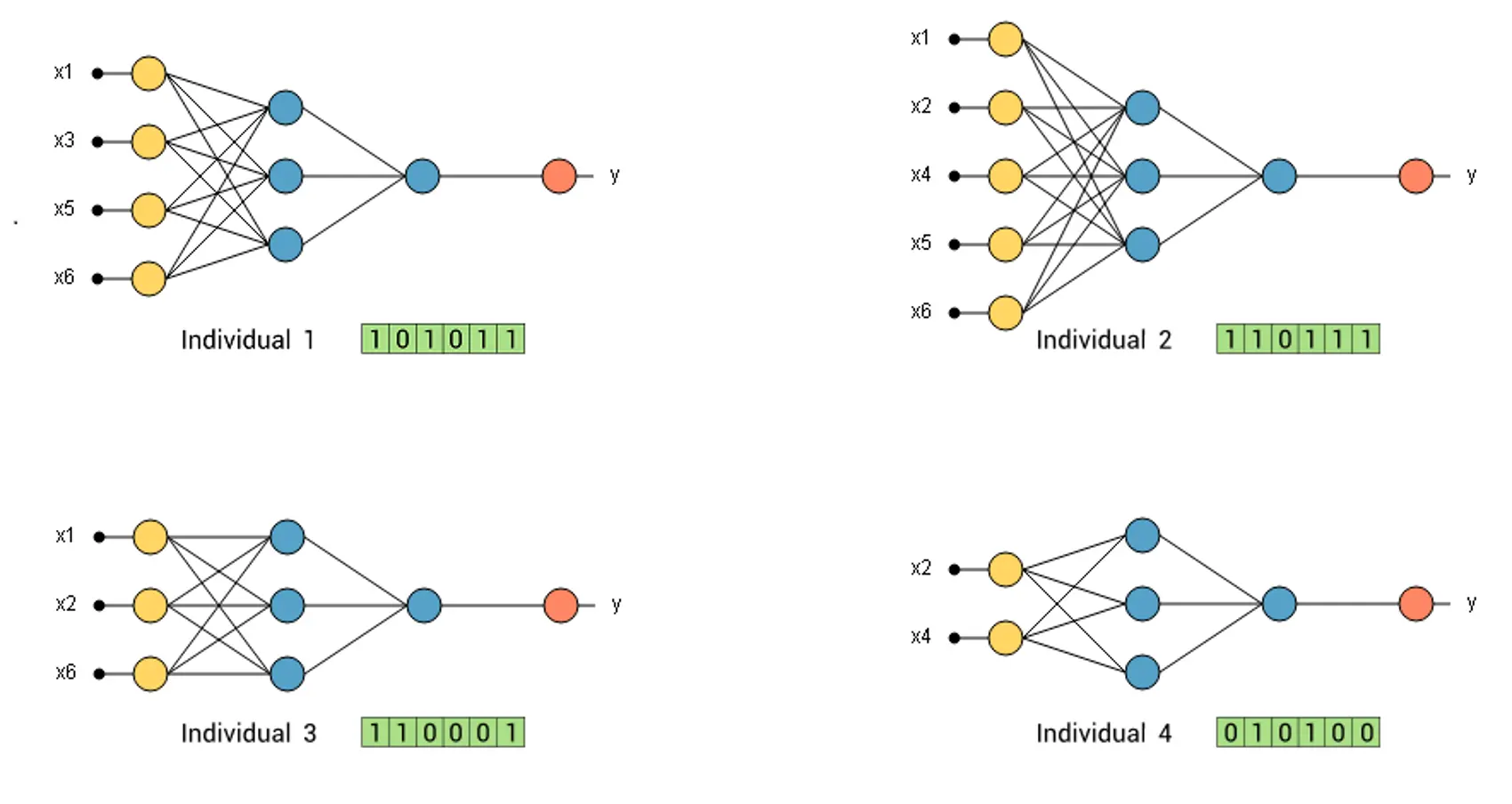As we can see, each individual is represented by 6 binary genes. Each positive gen means that the corresponding feature is included in the neural network.

## 2. Fitness assignment operator

After the initialization, we need to assign a fitness value to each individual in the population.

We train each neural network with the training instances and then evaluate their error with the selection instances.

A significant selection error means low fitness. Individuals with higher fitness are more likely to be selected for recombination.

The most used method for fitness assignment is known as a rank-based fitness assignment.

With this method, the selection errors of all the individuals are sorted.

Then, the fitness assigned to each individual only depends on its position in the individual's rank and not on the actual selection error.

The fitness value assigned to each individual with the rank-based method is:

$$\Phi(i) = k \cdot R(i) \qquad where \quad i = 1,...,N.$$

Here κ is a constant called selective pressure, and its value is fixed between 1 and 2. Higher selective pressure values make the fittest individuals have more probability of recombination. The parameter R(i) is the rank of individual i.

The following table depicts the selection error, the rank, and the corresponding fitness of each individual in our example.

Selection error Rank Fitness
Individual 1 0.9 1 1.5
Individual 2 0.6 3 4.5
Individual 3 0.7 2 3.0
Individual 4 0.5 4 6.0

Where we have chosen κ=1.5 to calculate the fitness value. Note that, for calculating the population fitness, we have trained 4 different neural networks .

We can plot the above fitness values in a pie chart. Here the area for each individual in the pie is proportional to its fitness. The following picture shows the fitness pie.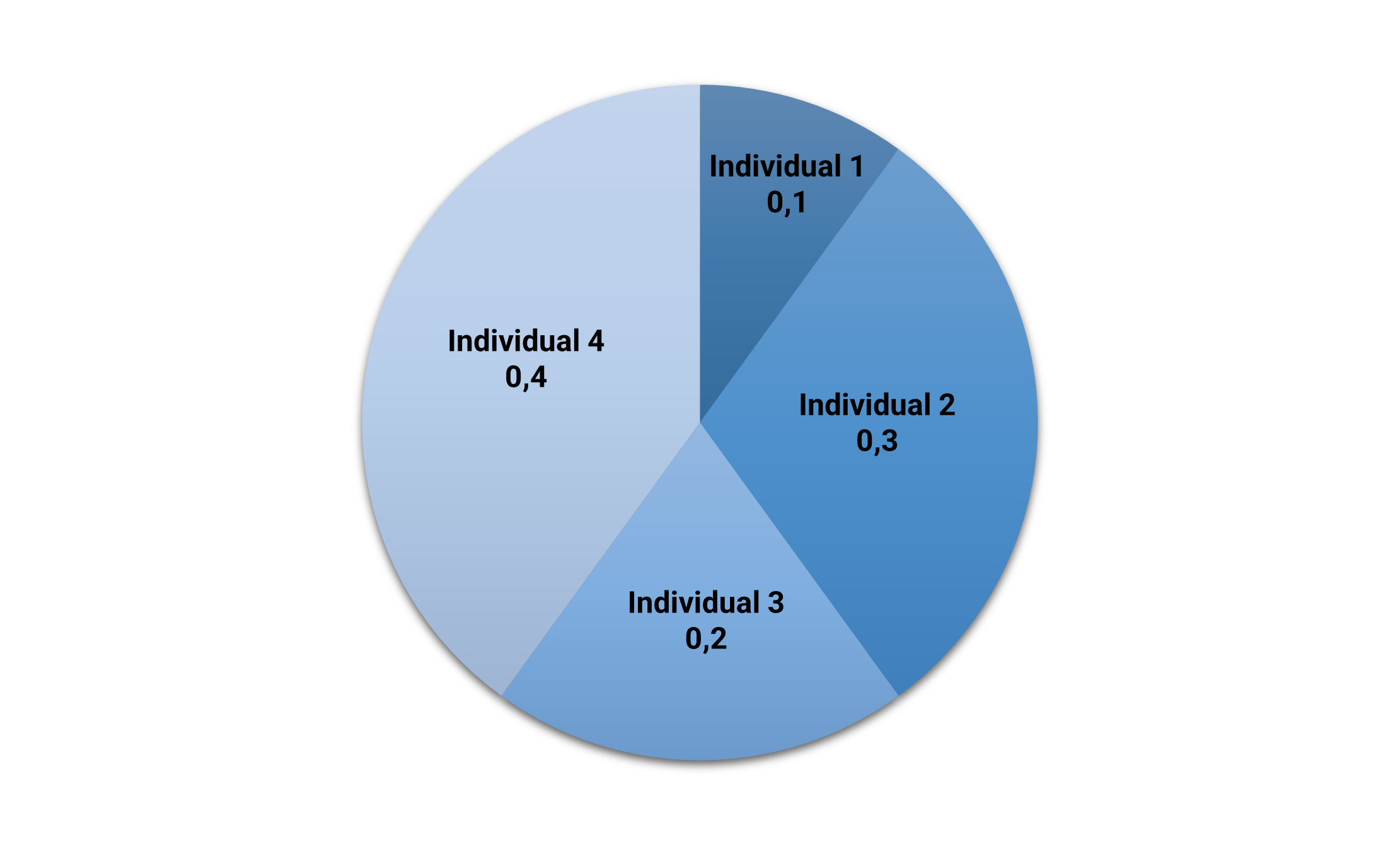As we can see, the fittest individual (#4) is the one who has the most significant area (40%). Conversely, the least fitted individual (#1) has the smallest area (10%).

## 3. Selection operator

After a fitness assignment has been performed, the selection operator chooses the individuals that recombine for the next generation.

The individuals most likely to survive are those more fitted to the environment. Therefore, the selection operator selects the individuals according to their fitness level. The number of selected individuals is N/2, being N the population size.

Elitism selection makes the fittest individuals survive directly for the next generation. The elitism size controls the number of directly selected individuals, and it is usually set to a small value (1,2,...).

One of the most used selection methods is the roulette wheel. This method places all the individuals on a roulette, with areas proportional to their fitness, as we saw above. Then, it turns the roulette and selects the individuals at random. The corresponding individual is selected for recombination.

The following figure illustrates the selection process for our example.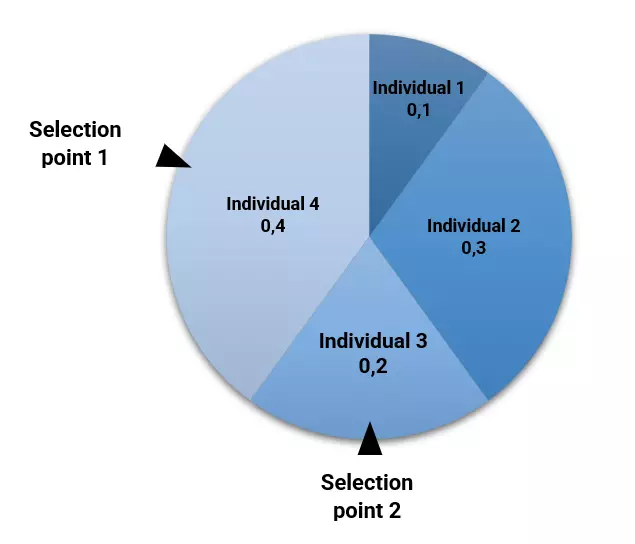In this case, neural network #4 has been selected by elitism, and #3 has been selected by the roulette wheel. Note that, although individual #2 has more fitness than #3, it has not been selected due to the stochastic nature of the genetic algorithm.

Here the number of selected individuals is half of the population size.

### 4. Crossover operator

Once the selection operator has chosen half of the population, the crossover operator recombines the selected individuals to generate a new population.

This operator picks two individuals randomly and combines their features to get four offspring for the new population until the new population is the same size as the old one.

The uniform crossover method decides whether each offspring's features come from one parent or another.

The following figure illustrates the uniform crossover method for our example.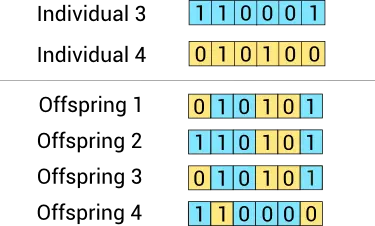Here we have generated four offspring from two parents. Some features of each neural network correspond to one ancestor and some other features to the other.

Here the population size remains constant.

## 5. Mutation operator

The crossover operator can generate offsprings that are very similar to the parents. This might cause a new generation with low diversity.

The mutation operator solves this problem by changing the value of some features in the offspring at random.

To decide if a feature is mutated, we generate a random number between 0 and 1. If the random number is lower than a value called the mutation rate, the operator flips that variable.

A standard value for the mutation rate is 1/m, where m is the number of features. We mutate one feature of each individual (statistically) with that value.

The following image shows the mutation of one offspring of the new generation.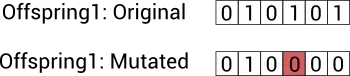As we can see, the fourth input of the neural network has been mutated.

At this point, we have a new population.

## Process and results

The whole fitness assignment, selection, recombination, and mutation process is repeated until a stopping criterion is satisfied. Each generation is likely to be more adapted to the environment than the old one.

The following figure shows the typical behaviour of the successive generations. The blue line represents the mean selection error of the individuals, and the red line represents the selection error of the best individual.As we can see, the mean selection error at each generation converges to a minimum value.

The solution to this process is the best individual ever. This corresponds to the neural network with the smallest selection error among all those we have analyzed.

In our example, the best individual is the following:The above neural network is the selected one for our application.

## Conclusions

Input selection is becoming a critical topic in machine learning. Many data sets contain a large number of features, so we need to select the most useful ones to be included in the neural network.

One of the most advanced methods to do that is the genetic algorithm.

Some advantages of genetic algorithms are the following:

• They usually perform better than traditional feature selection techniques.
• Genetic algorithms can manage data sets with many features.
• They don't need specific knowledge about the problem under study.
• These algorithms can be easily parallelized in computer clusters.

And some disadvantages are:

• Genetic Algorithms might be costly in computational terms. Indeed, the evaluation of each individual requires training a model.
• These algorithms can take a long time to converge since they are stochastic.

In conclusion, genetic algorithms can select the best subset of our model's variables, but they usually require much computation.

Neural Designer implements a more advanced genetic algorithm that the one described in this post. You can find it at the input selection section in the model selection panel.